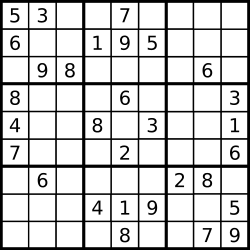# 36 Valid Sudoku – Easy

### Problem:

Determine if a Sudoku is valid, according to: Sudoku Puzzles – The Rules.

The Sudoku board could be partially filled, where empty cells are filled with the character '.'.A partially filled sudoku which is valid.

Note: A valid Sudoku board (partially filled) is not necessarily solvable. Only the filled cells need to be validated.

### Thoughts:

Just follow the basic rules for a valid Sudoku : No duplicate vertically, horizontal, squarely.

### Solutions:

``````public class Solution {
public boolean isValidSudoku(char[][] board) {
Set<Character> helper = new HashSet<Character>();
for (int i = 0;i < board.length; i ++) {
helper.clear();
for (int j = 0; j < board.length; j ++) {
if (process(helper, board[i][j]) == false)
return false;
}
}
for (int j = 0;j < board.length; j ++) {
helper.clear();
for (int i = 0; i < board.length; i ++) {
if (process(helper, board[i][j]) == false)
return false;
}
}
for(int i = 0; i<9; i+= 3){
for(int j = 0; j<9; j+= 3){
helper.clear();
for(int k = 0; k<9; k++){
if(!process(helper, board[i + k/3][ j + k%3]))
return false;
}
}
}

return true;
}
private boolean process(Set<Character> helper, char c) {
if (c == '.') {
return true;
}
if (helper.contains(c)) {
return false;
}